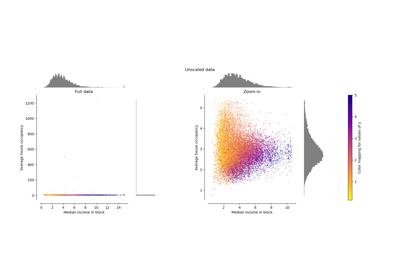# sklearn.preprocessing.MaxAbsScaler¶

class sklearn.preprocessing.MaxAbsScaler(*, copy=True)[source]

Scale each feature by its maximum absolute value.

This estimator scales and translates each feature individually such that the maximal absolute value of each feature in the training set will be 1.0. It does not shift/center the data, and thus does not destroy any sparsity.

This scaler can also be applied to sparse CSR or CSC matrices.

New in version 0.17.

Parameters:
copybool, default=True

Set to False to perform inplace scaling and avoid a copy (if the input is already a numpy array).

Attributes:
scale_ndarray of shape (n_features,)

Per feature relative scaling of the data.

New in version 0.17: scale_ attribute.

max_abs_ndarray of shape (n_features,)

Per feature maximum absolute value.

n_features_in_int

Number of features seen during fit.

New in version 0.24.

feature_names_in_ndarray of shape (n_features_in_,)

Names of features seen during fit. Defined only when X has feature names that are all strings.

New in version 1.0.

n_samples_seen_int

The number of samples processed by the estimator. Will be reset on new calls to fit, but increments across partial_fit calls.

maxabs_scale

Equivalent function without the estimator API.

Notes

NaNs are treated as missing values: disregarded in fit, and maintained in transform.

For a comparison of the different scalers, transformers, and normalizers, see examples/preprocessing/plot_all_scaling.py.

Examples

>>> from sklearn.preprocessing import MaxAbsScaler
>>> X = [[ 1., -1.,  2.],
...      [ 2.,  0.,  0.],
...      [ 0.,  1., -1.]]
>>> transformer = MaxAbsScaler().fit(X)
>>> transformer
MaxAbsScaler()
>>> transformer.transform(X)
array([[ 0.5, -1. ,  1. ],
[ 1. ,  0. ,  0. ],
[ 0. ,  1. , -0.5]])


Methods

 fit(X[, y]) Compute the maximum absolute value to be used for later scaling. fit_transform(X[, y]) Fit to data, then transform it. get_feature_names_out([input_features]) Get output feature names for transformation. get_params([deep]) Get parameters for this estimator. Scale back the data to the original representation. partial_fit(X[, y]) Online computation of max absolute value of X for later scaling. set_output(*[, transform]) Set output container. set_params(**params) Set the parameters of this estimator. Scale the data.
fit(X, y=None)[source]

Compute the maximum absolute value to be used for later scaling.

Parameters:
X{array-like, sparse matrix} of shape (n_samples, n_features)

The data used to compute the per-feature minimum and maximum used for later scaling along the features axis.

yNone

Ignored.

Returns:
selfobject

Fitted scaler.

fit_transform(X, y=None, **fit_params)[source]

Fit to data, then transform it.

Fits transformer to X and y with optional parameters fit_params and returns a transformed version of X.

Parameters:
Xarray-like of shape (n_samples, n_features)

Input samples.

yarray-like of shape (n_samples,) or (n_samples, n_outputs), default=None

Target values (None for unsupervised transformations).

**fit_paramsdict

Returns:
X_newndarray array of shape (n_samples, n_features_new)

Transformed array.

get_feature_names_out(input_features=None)[source]

Get output feature names for transformation.

Parameters:
input_featuresarray-like of str or None, default=None

Input features.

• If input_features is None, then feature_names_in_ is used as feature names in. If feature_names_in_ is not defined, then the following input feature names are generated: ["x0", "x1", ..., "x(n_features_in_ - 1)"].

• If input_features is an array-like, then input_features must match feature_names_in_ if feature_names_in_ is defined.

Returns:
feature_names_outndarray of str objects

Same as input features.

get_params(deep=True)[source]

Get parameters for this estimator.

Parameters:
deepbool, default=True

If True, will return the parameters for this estimator and contained subobjects that are estimators.

Returns:
paramsdict

Parameter names mapped to their values.

inverse_transform(X)[source]

Scale back the data to the original representation.

Parameters:
X{array-like, sparse matrix} of shape (n_samples, n_features)

The data that should be transformed back.

Returns:
X_tr{ndarray, sparse matrix} of shape (n_samples, n_features)

Transformed array.

partial_fit(X, y=None)[source]

Online computation of max absolute value of X for later scaling.

All of X is processed as a single batch. This is intended for cases when fit is not feasible due to very large number of n_samples or because X is read from a continuous stream.

Parameters:
X{array-like, sparse matrix} of shape (n_samples, n_features)

The data used to compute the mean and standard deviation used for later scaling along the features axis.

yNone

Ignored.

Returns:
selfobject

Fitted scaler.

set_output(*, transform=None)[source]

Set output container.

See Introducing the set_output API for an example on how to use the API.

Parameters:
transform{“default”, “pandas”}, default=None

Configure output of transform and fit_transform.

• "default": Default output format of a transformer

• "pandas": DataFrame output

• None: Transform configuration is unchanged

Returns:
selfestimator instance

Estimator instance.

set_params(**params)[source]

Set the parameters of this estimator.

The method works on simple estimators as well as on nested objects (such as Pipeline). The latter have parameters of the form <component>__<parameter> so that it’s possible to update each component of a nested object.

Parameters:
**paramsdict

Estimator parameters.

Returns:
selfestimator instance

Estimator instance.

transform(X)[source]

Scale the data.

Parameters:
X{array-like, sparse matrix} of shape (n_samples, n_features)

The data that should be scaled.

Returns:
X_tr{ndarray, sparse matrix} of shape (n_samples, n_features)

Transformed array.

## Examples using sklearn.preprocessing.MaxAbsScaler¶Compare the effect of different scalers on data with outliers

Compare the effect of different scalers on data with outliers